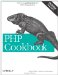# Recipe 3.10. Adding to or Subtracting from a Date

#### 3.10.1. Problem

You need to add or subtract an interval from a date.

#### 3.10.2. Solution

Depending on how your date and interval are represented, use strtotime( ) or some simple arithmetic.

If you have your date and interval in appropriate formats, the easiest thing to do is use strtotime( ), as in Example 3-24.

##### Calculating a date interval with strtotime( )

 ``

If your date is an epoch timestamp and you can express your interval in seconds, subtract the interval from the timestamp, as in Example 3-25.

##### Calculating a date interval with epoch timestamps

 ``

#### 3.10.3. Discussion

Using strtotime( ) is good for intervals that are of varying lengths, such as months. If you can't use strtotime( ), convert your date to an epoch timestamp and add or subtract the appropriate interval in seconds. This is mostly useful for intervals of a fixed time, such as days or weeks. Example 3-26 adds seven days' worth of seconds to a timestamp.

##### Another date interval with epoch timestamps

 ``

Using this method, however, you can run into problems if the endpoints of your interval are on different sides of a DST switch. In this case, one of your fixed-length days isn't 86,400 seconds long; it's either 82,800 or 90,000 seconds long, depending on the season.

Recipe 3.5 for finding the difference between two dates in elapsed time; Recipe 3.6 for finding the difference between two dates in Julian days; documentation on strtotime( ) at http://www.php.net/strtotime.PHP Cookbook: Solutions and Examples for PHP Programmers
ISBN: 0596101015
EAN: 2147483647
Year: 2006
Pages: 445

Similar book on Amazon